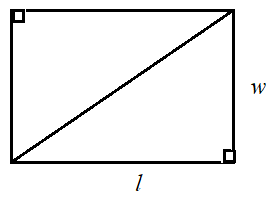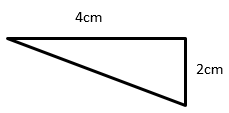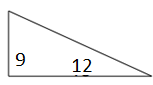# Finding the area of a right triangle or its corresponding rectangle

Area of a right triangle

Consider a rectangle of length l units and width w units. Let us draw a diagonal and cut out the rectangle. Let us also cut along the diagonal to form two right triangles.It is found that the two right triangles have the same size and area and are congruent. So the area of each right triangle is half the area of the rectangle.

Area of right triangle = $\frac{1}{2}$ area of rectangle = $\frac{1}{2}$ l × w

The length l of the rectangle is the base b of the right triangle and the width w of the rectangle is the height h of the right triangle.

So area of right triangle = $\mathbf{\frac{1}{2}}$ l × w = $\mathbf{\frac{1}{2}}$ b × h

Area of a right triangle is half the area of its corresponding rectangle

Find the area of following right triangle and its corresponding rectangle.### Solution

Step 1:

Area of Right Triangle = $\frac{1}{2}$ × b × h; b = base = 4; h = height = 2

Step 2:

Area of Right Triangle = $\frac{1}{2}$ × 4 × 2 = 4 square cm

Area of corresponding rectangle = b × h = 4 × 2 = 8 square cm

Find the area of following right triangle and its corresponding rectangle.### Solution

Step 1:

Area of Right Triangle = $\frac{1}{2}$ × b × h; b = base = 12; h = height = 9

Step 2:

Area of Right Triangle = $\frac{1}{2}$ × 12 × 9 = 54 square units

Area of corresponding rectangle = b × h = 12 × 9 = 108 square units